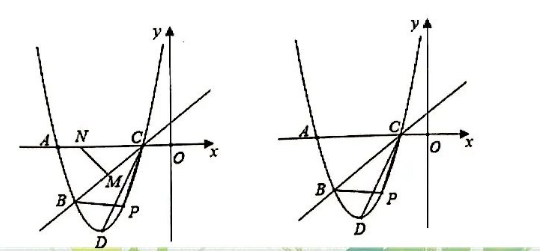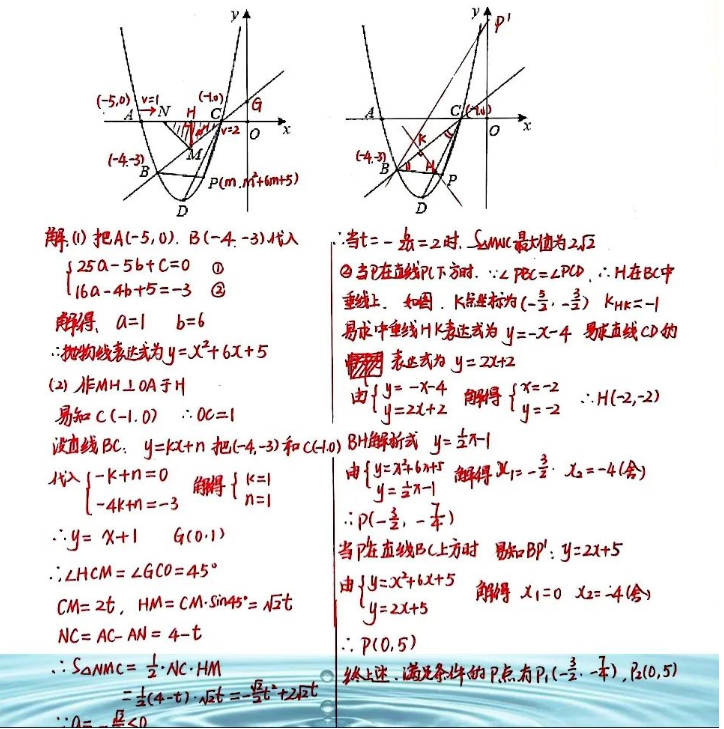(1)求该抛物线的表达式;
(2) 点 $P$ 为该抛物线上一动点 (与点 $B 、 C$ 不重合), 设点 $P$ 的横坐标为 $m$.
(1)点 $M$ 从点 $C$ 出发在线段 $C B$ 上以每秒 2 个单位长度的速度向点 $B$ 运动, 同时点 $N$ 从点 $A$ 出发以每秒 1 个单 位长度的速度向点 $C$ 运动, 当其中一个点到达终点时, 另外一个点也停止运动, 设运动时间为 $t$ 秒, 求运动时 间为多少时, $\triangle C M N$ 的面积最大, 并求出最大面积;
(2)该扐物线上是否存在点 $P$, 使得 $\angle P B C=\angle B C D$ ? 若存在, 求出所有点 $P$ 的坐标; 若不存在, 请说明理由.【答案】